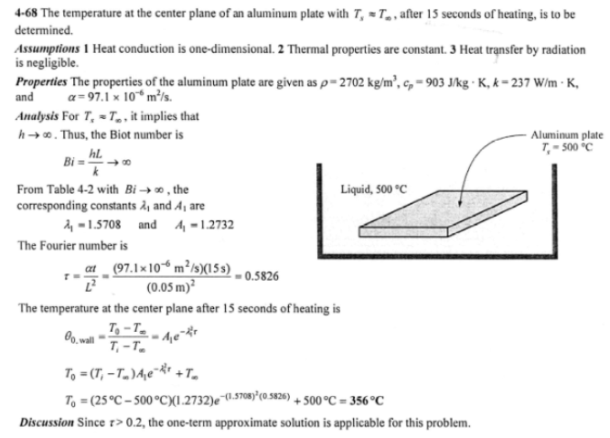heat and mass transfer problems engineering equations heat and mass transfer school homework engineering heat and mass transfer formulas heat and mass transfer solutions to heat and mass transfer problems full solution engineering problem solution heat and mass transfer math problems engineering equations heat and mass transfer school homework engineering solutions to heat and mass transfer formulas heat problems mass problem solutions to transfer problems full solution heat and mass transfer
heat and mass transfer problems engineering equations heat and mass transfer school homework engineering heat and mass transfer formulas heat and mass transfer solutions to heat and mass transfer problems full solution engineering problem solution heat and mass transfer math problems engineering equations heat and mass transfer school homework engineering solutions to heat and mass transfer formulas heat problems mass problem solutions to transfer problems full solution heat and mass transfer
Highalphabet Home Page heat and mass transfer problem solutions Heat and Mass Transfer Page
A 10-cm thick aluminum plate (rho=2702 kg/m^3, c_p=903 J/kg K, k=273 W/mK, and alpha=97.1x10^-6 m^2/s) is being heated in liquid with temperature of 500 C. The aluminum plate has a uniform initial temperature of 25 C. If the surface temperature of the aluminum plate is approximately the liquid temperature, determine the temperature at the center plane of the aluminum plate after 15 seconds of heating.A 10-cm thick aluminum plate (rho=2702 kg/m^3, c_p=903 J/kg K, k=273 W/mK, and alpha=97.1x10^-6 m^2/s) is being heated in liquid with temperature of 500 C. The aluminum plate has a uniform initial temperature of 25 C. If the surface temperature of the aluminum plate is approximately the liquid temperature, determine the temperature at the center plane of the aluminum plate after 15 seconds of heating.